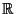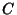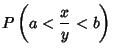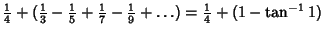## Real Number

The set of all Rational and Irrational numbers is called the real numbers, or simply the reals,'' and denoted. The set of real numbers is also called the Continuum, denoted.

The real numbers can be extended with the addition of the Imaginary Number i, equal to. Numbers of the form, whereandare both real, are then called Complex Numbers. Another extension which includes both the real numbers and the infinite Ordinal Numbers of Georg Cantoris the Surreal Numbers.

Pick two real numbersandat random inwith a Uniform Distribution. What is the Probabilitythat, wheredenotes Nint, the nearest Integer to, is Even? The answer may be found as follows (Putnam Exam).(1)(2)

Plouffe's Inverse Symbolic Calculator'' includes a huge database of 54 million real numbers which are algebraically related to fundamental mathematical constants and functions.

See also Complex Number, Continuum, i, Imaginary Number, Integer Relation, Rational Number, Real Part, Surreal Number

References

Plouffe, S. Inverse Symbolic Calculator.'' http://www.cecm.sfu.ca/projects/ISC/.

Plouffe, S. Plouffe's Inverter.'' http://www.lacim.uqam.ca/pi/.

Putnam Exam. Problem B-3 in the 54th Putnam Exam.